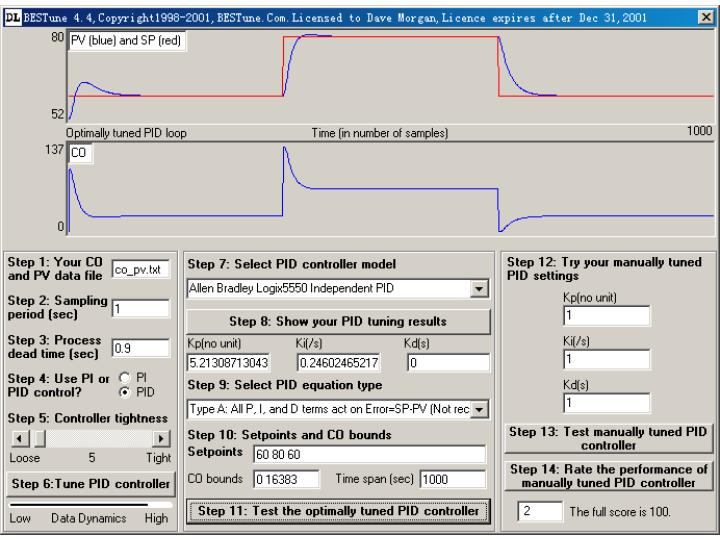# Three Types of PID Equations

Consider the "Allen Bradley Logix5550 Independent PID equation":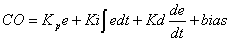(1)

where CO the controller output, e=SP-PV, SP the setpoint, PV the process variable. Differentiating both sides gives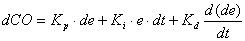(2)

Using difference to approximate the differential we get discrete PID equation in type A.

Type A:where T is the sampling period (also called loop update time). Type A is called textbook PID and many experienced engineers dislike it. This is because the D-term contains setpoint and changes in setpoint may cause an unwanted change in CO. So they remove setpoint from D-term and get type B.

Type B: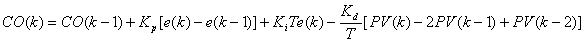Many industrial PID controllers are of type B (e.g. Allen Bradley PLCs). However, if we remove setpoint from both P-term and D-term, we get a still better PID equation type C.

Type C: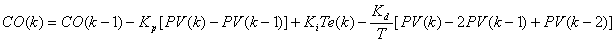In all these three types CO should be within the user specified range, i.e., CO should be equal to its upper limit if it is larger than its upper limit according to the above PID equations, and CO should be equal to its lower limit if it is smaller than its lower limit according to the PID equations. Unfortunately this is often forgotten when designing a PID controller, which will typically cause the so called "wind-up" problem if the controller is tightly tuned.

Some industrial PID controllers allow users to select one of the three types. Whenever possible, type C should be used. If type C is not available, then use type B. If you are in a difficult situation where you have to use type A, then reduce the tightness of your PID controller until there is no overshoot (see Figure 4 below). BESTune has the feature to allow you to specify controller tightness before tuning your PID controllers. A further measure to overcome the difficulty brought by type A is to let the D-term be zero, i.e., in step 4 of BESTune GUI select "PI" to make your controller a PI controller.

For the same PID parameters Kp, Ki, and Kd, the following figures show that the performance of type C is the best and type A is the worst. To improve the performance of type A, we have to sacrifice the controller tightness (This will lead to a longer settling time, see Figure 4). If you want to try to tune this PID loop, you can get the CO and PV measurements and all the necessary info from http://bestune.50megs.com/CO_PV.htm. If you want to know how to design a practical type C PID controller, it is strongly recommended that you take a look at http://bestune.50megs.com/piddesign.htm.

If you look at Equation (2), the fact that the setpoint should be removed from the P-term and D-term is obvious because otherwise the P-term will become an infinity (a Dirac function) and so will the D-term if there is a step change in SP. Therefore, the traditional PID equations (1) and (2) should be changed to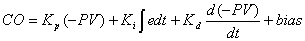and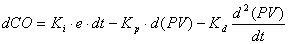Type A may cause overshoot if the setpoint is not second order differentiable. Type B may cause overshoot if the setpoint is not first order differentiable. In case overshoot should be strictly avoided, Type C is the best choice. Type B and type A should be avoided.

BESTune, June 4, 2001

Figure 1: Performance of Type C (best).Figure 2: Performance of Type B (worse than type C better than type A).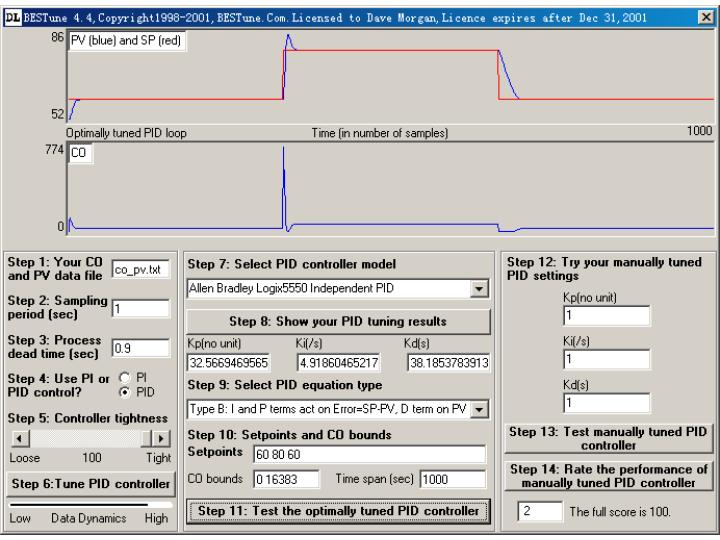Figure 3: Performance of Type A (worst).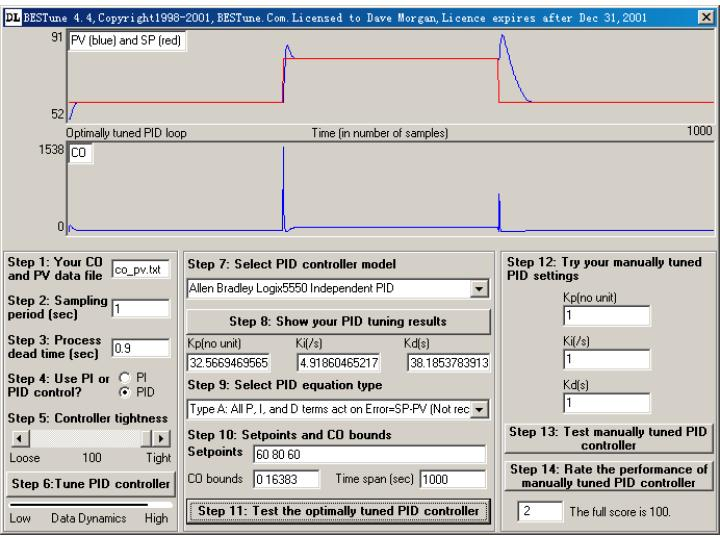Figure 4: Performance of Type A (reduced tightness and longer settling time).